# Alternion

(diff) ← Older revision | Latest revision (diff) | Newer revision → (diff)

A hypercomplex number. Alternions may be considered as a generalization of the complex numbers, double numbers (cf. Double and dual numbers) and quaternions. The algebraof alternions of orderand of indexis an algebra of dimension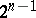over the field of real numbers, with unit element 1 and a system of generators, in which the multiplication satisfies the formula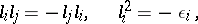where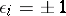, the value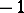occurstimes and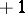occurs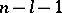times, respectively. A base of the algebra is formed by the unit element and by elements of the form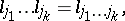where. In this base any alternioncan be written as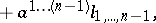where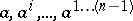are real numbers. The alternion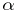conjugate to the alternionis defined by the formula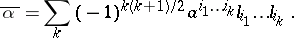The following equalities holdThe productis always a positive real number; the quantity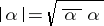is called the modulus of the alternion. If the number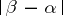is taken as the distance between two alternionsand, then the algebras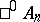and,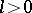, are isometric to the Euclidean space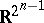and the pseudo-Euclidean spaces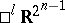, respectively. The algebrais isomorphic to the field of real numbers;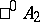is isomorphic to the field of complex numbers;is isomorphic to the algebra of double numbers;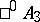is isomorphic to the skew-field of quaternions; andandare isomorphic to the so-called algebras of anti-quaternions. The elements ofare the so-called Clifford numbers. The algebra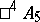was studied by P. Dirac in the context of the spin of an electron.

The algebras of alternions are special cases of Clifford algebras (cf. Clifford algebra).

How to Cite This Entry:
Alternion. Encyclopedia of Mathematics. URL: http://encyclopediaofmath.org/index.php?title=Alternion&oldid=15232
This article was adapted from an original article by N.N. Vil'yams (originator), which appeared in Encyclopedia of Mathematics - ISBN 1402006098. See original article